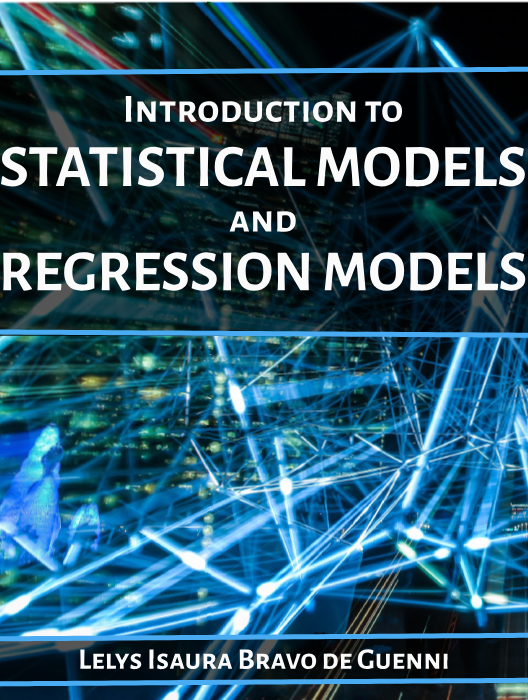# Introduction to Statistical Methods and Regression Models

Lead Author(s): Lelys Bravo de Guenni

This book is an introduction to the main methods used in Statistical Inference and Regression Analysis, using real life examples and a statistical package outputs.

# Introduction to Statistical Methods and Regression Models

Lelys Bravo de Guenni, PhD

## Chapter 1: Introduction to Statistical Thinking

• Questions to Chapter 1

## Chapter 2: Descriptive Statistics and Sampling Distributions

• Part I: Descriptive Statistics
• Part II: Sampling Distributions
• Question to Chapter 2

## Chapter 3: Basic Statistical Inference

• 3.1 Point Estimation
• 3.2 Interval Estimation
• 3.3 Hypothesis Testing
• Questions to Chapter 3

## Chapter 4: Correlation Analysis

• Part I: Definition of the Correlation Coefficient
• Part II: Hypothesis testing and confidence intervals for the correlation coefficient ρ
• Questions to Chapter 4

## Chapter 5: Simple Linear Regression Analysis

• Part I: Model assumptions and least-square estimation
• Part II: Inference on the linear  regression equation
• Part III. The Analysis of Variance Table: Assessing the appropriateness of the straight-line regression
• Questions to Chapter 5

## Chapter 6: Multiple Regression Analysis

• Part I: Examples and Analysis of Variance for the Multiple Regression equation
• Part II: Statistical Inference in Multiple Regression
• Part III. Multiple correlation coefficient, partial correlation and multiple partial correlation coefficient
• Questions to Chapter 6

## Chapter 7: Dummy variables in regression and straight-line comparisons

• 7.1 Dummy Variables in Regression
• 7.2 Comparing two straight-lines
• Questions to Chapter 7

## Chapter 8: Regression Diagnostics and Best Model Selection

• Part I: Regression Diagnostics
• Part II: Selecting the Best Regression Equation
• Questions to Chapter 8